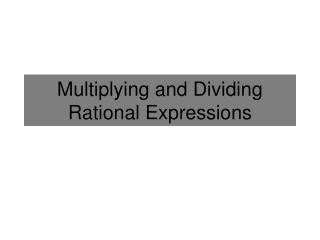DownloadDownload PresentationMultiplying and Dividing Rational Expressions

# Multiplying and Dividing Rational Expressions

Télécharger la présentation## Multiplying and Dividing Rational Expressions

- - - - - - - - - - - - - - - - - - - - - - - - - - - E N D - - - - - - - - - - - - - - - - - - - - - - - - - - -
##### Presentation Transcript

1. Multiplying and Dividing Rational Expressions

2. Multiplying and Dividing Fractions Multiply Numerators Multiply: Multiply Denominators Divide: Multiply by the reciprocal (flip) Remember to Simplify!

3. Example 1 • Simplify the following expressions: The techniques we use to simplify a fraction without variables (Finding the greatest common FACTOR) is the same we will use to simplify fractions with variables.

4. Example 2 These are just the values of x that make the expression undefined. Simplify: Half the work is done. It is already factored. Combine the fractions by multiplying Rewrite any factors if they are raised to a power Cancel common factors

5. Example 3 These are just the values of x that make the expression undefined. Simplify: Multiply by the reciprocal (Flip the fraction) Can NOT cancel since its not in factored form. Factor Make sure to Factor Completely Cancel common factors

6. OR

7. Example 3 These are just the values of x that make the expression undefined. Simplify: Can NOT cancel since its not in factored form. Multiply by the reciprocal (Flip the fraction) Almost the same as x – 5 Factor Make sure to Factor Completely TRICK: Factor out -1 to make it the same. Cancel common factors